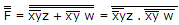# Electronics and Communication Engineering - Digital Electronics - Discussion

31.

Minimum number of 2-input NAND gates required to implement the function F = (x + y) (Z + W) is

 [A]. 3 [B]. 4 [C]. 5 [D]. 6

Explanation:

F = (x + y) (z + w) = xy.(z + w)

= xyz + xyw

=minimum no. of 2 input NAND gate.

 Shiavnjali said: (Jun 16, 2015) Any one can explain in detail?

 Mangesh said: (Jan 20, 2016) Why are we taking double bar?

 Kruthi said: (Jun 27, 2016) We can replace 1 with double bar.

 Riya said: (Sep 3, 2016) To get the simplified expression in terms of product form we take double bar on both sides.(the double bar doesn't change the value i.e.,F''=F) If, F'' = ((X' + Y')(Z = W))'', F = (X'Z + X'W + Y'Z + Y'W)'', F = ((X' + Y')' + (Z + W)')', F = (X Y + Z'W')', F = (X Y)' (Z + W, F = (X Y)'Z + (X Y)'W. By using four NAND gate we can implement the final expression.

 Gaurang said: (Sep 24, 2016) Use DOT conversion rule, so we can convert F = (x' + y') (z + w) = xy + z'w'. Now implement this using two input NAND gates. Note: Use NAND as inverter to get z' and w' The answer should be 5.

 Tulasiram said: (Mar 17, 2017) (x'+y')(z+w)--->xor gate. 4 nand gates are required.

 Jbel said: (Mar 20, 2017) (x' + y') (z + w) --- overall, AND function - to make NAND -> DOUBLE COMPLEMENT { [ (x' + y') (z + w) ]' }' -- then simplify terms in parenthesis: FACTOR OUT Negation (remember De Morgans). { [ (x y)' (z' w')' ]' }' (xy)' - 1 NAND z' - 1 NAND (complementation: SHORT two inputs) w' - 1 NAND (complementation: SHORT two inputs) (z'w')' - 1 NAND [ (x y)' (z' w')' ]' - 1 NAND for the overall gate (carries all operations) { [ (x y)' (z' w')' ]' }' - LAST NAND for complement, since original term has AND overall function. Overall, 6 NAND gates. Well, not including INVERTERS, that would be 4.

 Jagannath said: (Aug 27, 2017) F=(x'+y')(w+z)=(xy)'(w+z) now 1st take a NAND gate with the input X,Y that will produce (xy)'=k. Let assume then take another two NAND gate which inputs are K ,W and K,Z then take another NAND gate with the input of those outputs that will produce the desire output.

 Jaan said: (Oct 12, 2017) For implementing I think we should not alter the given Boolean equation and according to the procedure it requires 6 NAND gates.

 Rajkumar said: (Apr 22, 2019) 5 two input Nand gates.

 Apoorva said: (Aug 19, 2019) Can someone explain the same problem with circuit concept?

 Harshita Sharma said: (Mar 11, 2020) F=(x'+y').(Z+W) F=(xy)'.(Z+W) F=(xy)'Z + (xy)'W NAND GATE 1: INPUT 1 = x INPUT 2 = y OUTPUT = (xy)' Take this output as K => [K = (xy)'] NAND GATE 2: INPUT 1 = Z INPUT 2 = K OUTPUT = [(xy)'z]' Take this output as L => L= [(xy)'z]' NAND GATE 3: INPUT 1 = W INPUT 2 = K OUTPUT = [(xy)'W]' Take this output as M => M = [(xy)'W]' NAND GATE 4: INPUT 1 = L INPUT 2 = M OUTPUT = [ ((xy)'W)' . ((xy)'Z)' ]' Using De Morgan's Law (A.B)' = A' + B' (A + B)' = (A)' . (B)' OUTPUT = { [(xy)'W]' }' + { [(xy)'Z]' }' OUTPUT = (xy)'W + (xy)'Z This output is equal to F = (xy)'Z + (xy)'W I Hope, this solution will give clarity

 Vishnu said: (May 8, 2021) As far as time is concerned u won't get that much time to solve this question here is the shortcut. What is nand====(xy)'==x'+y'. Sso here is shortcut==(x'+y')(w+z)=(xy)'(w'z')'==1nand for (xy)' + 1 nand for (w'z')' + 1 nand to convert z' to z +1 nand to convert w' to w as we r not alloweded to use not in the circuit. Hope it helped you guys.